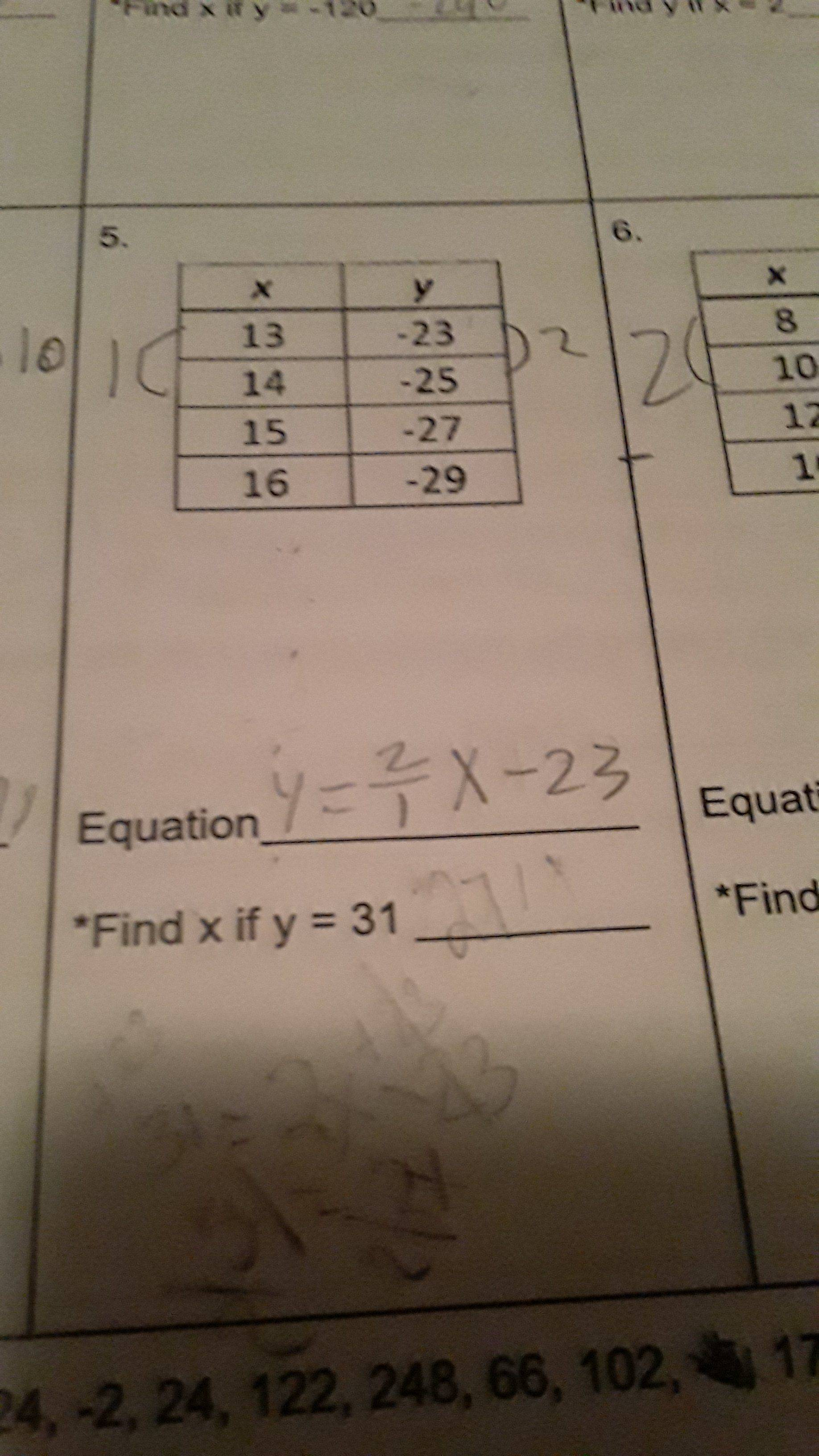# How to do this problem

• MHB
• Sarahq

#### SarahqHi Sarahq, and welcome to MHB!

For your Problem 5, you should notice that as $X$ increases by $1$, $Y$ decreases by $2$. So the equation between $X$ and $Y$ should start as $Y = \dfrac{-2}1X \ldots$. You should then be able to find the constant term to complete the equation.

Hi Sarahq,

The formula for linear equations is
$y = mx+b$
m = slope and b = shift on the y-axis.

The following formula is used to calculate the slope m
$m = \frac{y_2-y_1}{x_2-x_1}$
(here -2/1 = -2)
Use x-, y-values and m to calculate b:
$-23 = -2 * 13 + b$
solve for b:
$-23 = -26 + b | +26$
$3 = b$
so:
$y = -2x + 3$
Now just set y = 31 and solve for x.